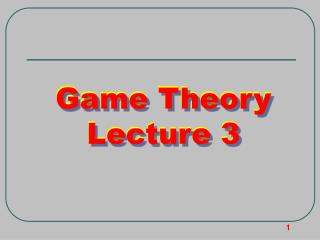# Game Theory Lecture 3 - PowerPoint PPT PresentationDownload PresentationGame Theory Lecture 3

Presentation Description
Download Presentation## Game Theory Lecture 3

- - - - - - - - - - - - - - - - - - - - - - - - - - - E N D - - - - - - - - - - - - - - - - - - - - - - - - - - -
##### Presentation Transcript

1. Game Theory Lecture 3 Game Theory Lecture 3

2. 0 6 1 1/6 4 5 3 2 1 1 1 1 1 1 N.S. S D,W D,W D,W D,W D,W D,W L,W 2 2 2 2 2 W,D W,D W,D W,D W,D W,L 1 1 1 1 D,W D,W D,W D,W L,W 2 2 2 W,D W,D W,D W,L 1 1 D,W D,W L,W 2 W,D W,L

3. A Lottery 2 2 2 W,D W,D W,D S. W,L 1 1 N.S. S D,W D,W L,W N.S. 2 N.S. W,D W,D S W,L W,L

4.  A Lottery consider the lottery ? for S. N.S. assume that

5. 2 2 2 W,D W,D W,D S. W,L 1 1  N.S. S D,W D,W L,W N.S. 2 W,D W,L

6. N.S. 2 2 2 S. W,D W,D W,D W,L W,L 1 1 N.S. S D,W D,W L,W L,W 2 W,D W,D W,L

7.  N.S. ? S.

8. von Neumann - Morgenstern utility functions A consumer has preferences over a set of prizes and preferences over the set of all lotteries over the prizes

9. 4. if then:

10. 5.

11. we now look for a utlity function representing the preferences over the lotteries

12. take a lottery: Replace each prize with an equivalent lottery

13. define: the expected utility of the lottery clearly U represents the preferences on the lotteries

14.  <

15. If is a vN-M utility function then is a vN-M utility function iff A utility function on prizes is called a von Neumann - Morgenstern utility function if the expected utility function : represents the preferences over the lotteries. i.e. if U is a utility function for lotteries.

16. If is a vN-M utility function then is a vN-M utility function iff • It is easy to show that if u( ) is a vN-M utility function • then so is au( )+b a>0 2. Let v() be a vN-M utility function. Choose a>0 ,b s.t.

17. since f( ) is a vN-M utility function, and since for all j It follows that: But by the definition of f( ) hence:

18. Neumann Janos John von Neumann 1903-1957 Oskar Morgenstern 1902-1976 Kurt Gödel

19. Information Sets and Simultaneous Moves 1 2 2

20. Some (classical) examples of simultaneous games Prisoners’ Dilemma +6

21. Free Rider (Trittbrettfahrer)

22. Some (classical) examples of simultaneous games Prisoners’ Dilemma The ‘D strategy dominates the C strategy

23. Strategy s1strictly dominates strategys2 if for all strategies tof the other player G1(s1,t)> G1(s2,t)

24. Successive deletion of dominated strategies X X Nash Equilibrium (saddle point)